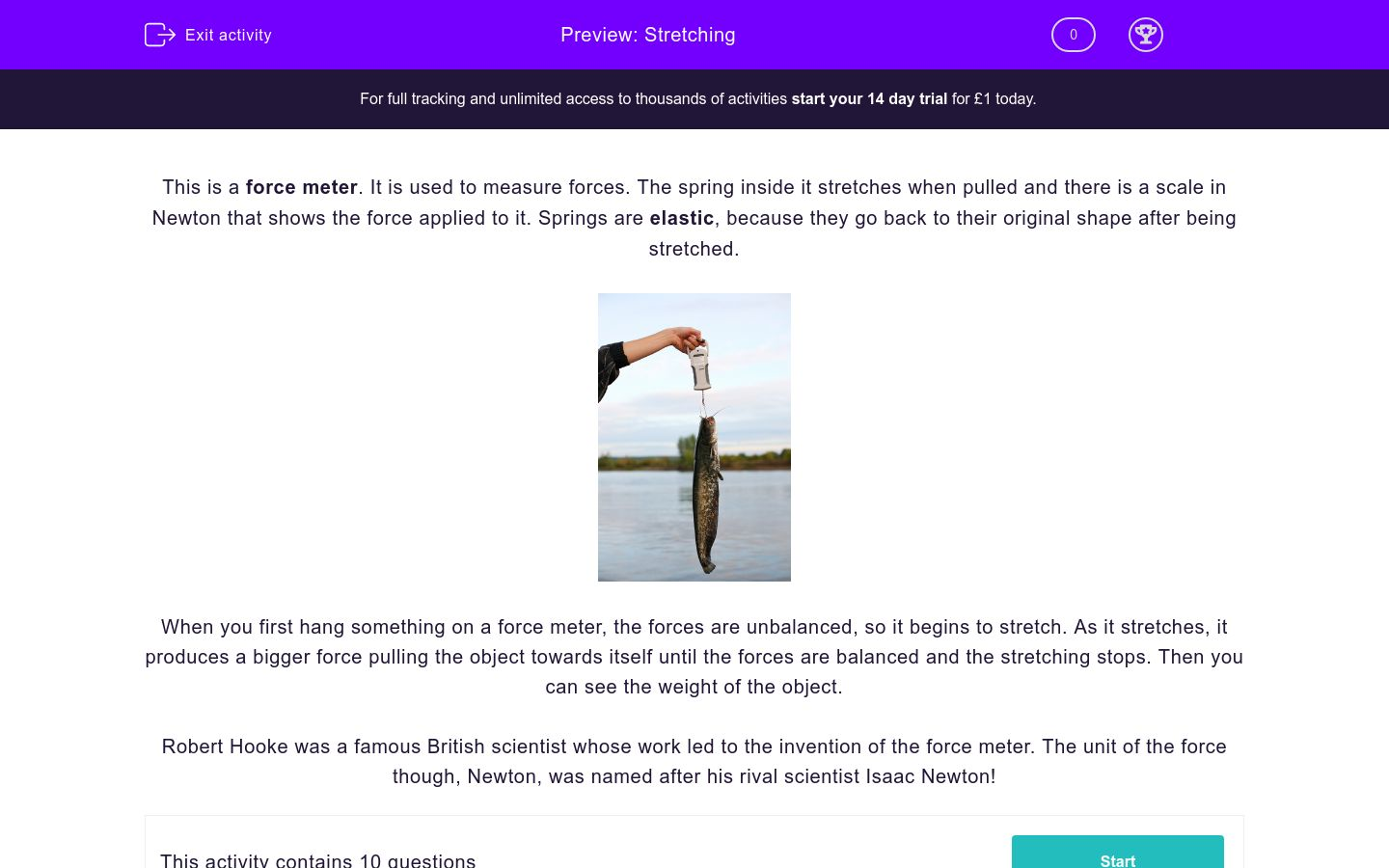# Stretching

In this worksheet, students will learn how things stretch.Key stage:  KS 3

Curriculum topic:   Physics: Motion and Forces

Curriculum subtopic:   Forces

Difficulty level:### QUESTION 1 of 10

This is a force meter. It is used to measure forces. The spring inside it stretches when pulled and there is a scale in Newton that shows the force applied to it. Springs are elastic, because they go back to their original shape after being stretched.When you first hang something on a force meter, the forces are unbalanced, so it begins to stretch. As it stretches, it produces a bigger force pulling the object towards itself until the forces are balanced and the stretching stops. Then you can see the weight of the object.

Robert Hooke was a famous British scientist whose work led to the invention of the force meter. The unit of the force though, Newton, was named after his rival scientist Isaac Newton!

Write the missing word to complete this sentence.

The instrument that is used to measure forces is called a _____________ .

Complete this sentence.

What stretches inside a force meter? A ___________ .

What numerical scale do we see on a force meter?

metre

Newton

force

How would you describe the forces when you first hang something on a force meter?

balanced

unbalanced

How would you describe things that stretch and then go back to their original shape, such as a spring?

elastic

rigid

What happens to the force caused by a spring as it stretches?

it increases

it decreases

it stays the same

How would you describe the forces when the spring stops stretching?

balanced

unbalanced

Complete this sentence.

The weight of an object hanging on a force meter should be measured ________________________.

as soon as you hang it

as soon as the spring stops stretching

while the spring is stretching

Which scientist's work led to the invention of the force meter?

Robert Newton

Isaac Newton

Robert Hooke

Whose scientist's name was used as the unit for forces?

Walter Weight

Robert Hooke

Isaac Newton

• Question 1

Write the missing word to complete this sentence.

The instrument that is used to measure forces is called a _____________ .

force meter
EDDIE SAYS
Force meters are used to measure forces.
• Question 2

Complete this sentence.

What stretches inside a force meter? A ___________ .

spring
EDDIE SAYS
Force meters have a spring inside, which stretches and helps to measure the force.
• Question 3

What numerical scale do we see on a force meter?

Newton
EDDIE SAYS
The scale is in Newtons; force meters are also called Newton meters.
• Question 4

How would you describe the forces when you first hang something on a force meter?

unbalanced
EDDIE SAYS
The forces are unbalanced when you first hang something on a force meter.
• Question 5

How would you describe things that stretch and then go back to their original shape, such as a spring?

elastic
EDDIE SAYS
Elastic things stretch and then go back to their original shape.
• Question 6

What happens to the force caused by a spring as it stretches?

it increases
EDDIE SAYS
The force caused by the spring increases as it stretches. This is because as the weight causes it to stretch (deform), the spring pulls against the weight with an incresing force until they are balanced (reach equilibrium).
• Question 7

How would you describe the forces when the spring stops stretching?

balanced
EDDIE SAYS
When the spring stops stretching the forces are balanced.
• Question 8

Complete this sentence.

The weight of an object hanging on a force meter should be measured ________________________.

as soon as the spring stops stretching
EDDIE SAYS
A reading should be taken from a force meter as soon as the spring stops stretching.
• Question 9

Which scientist's work led to the invention of the force meter?

Robert Hooke
EDDIE SAYS
Robert Hooke's work led to the invention of the force meter.
• Question 10

Whose scientist's name was used as the unit for forces?

Isaac Newton
EDDIE SAYS
The unit for forces was named after Isaac Newton.
---- OR ----

Sign up for a £1 trial so you can track and measure your child's progress on this activity.

### What is EdPlace?

We're your National Curriculum aligned online education content provider helping each child succeed in English, maths and science from year 1 to GCSE. With an EdPlace account you’ll be able to track and measure progress, helping each child achieve their best. We build confidence and attainment by personalising each child’s learning at a level that suits them.

Get started